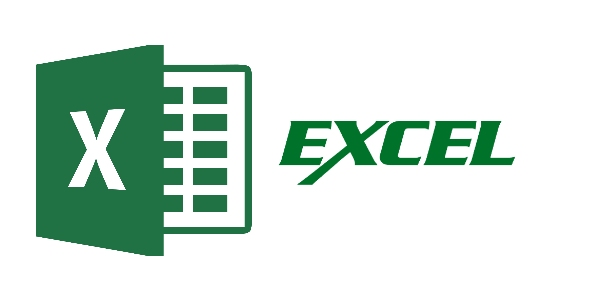# Excel 2007 (Pre Test)

15 Questions | Total Attempts: 58Settings• 1.
Is addition of rows and columns is possible beyond defined limit
• A.

Yes

• B.

No

• C.

None of the above

• 2.
Select the most appropriate amongst the following
• A.

Goal Seek can be used conditionally to solve scenarios based on multiple constraints

• B.

Goal Seek can be used conditionally to solve scenarios based on single constraints

• C.

Goal Seek is not an excel command

• D.

Iterations must be enabled in order to use Goal Seek

• 3.
Which of the following function syntax is correct?
• A.

=Vlookup(A1,\$A\$1:\$B\$12,"First Name",0)

• B.

=Vlookup(A1:A101, \$A\$1:\$B\$12,2,0)

• C.

=Vlookup(A1, \$A\$1:\$B\$12,1,0)

• D.

None of the above

• 4.
A column chart is made out of data present in A1:B5. For a new data added in A6 and B6, the chart has to be redesigned to include the new record
• A.

There is no feature in excel to solve this problem

• B.

This problem can be easily solved by converting your data into a list/ table range

• C.

The chart will get auto updated with the new records, if the data is refreshed

• D.

Column chart does not support new records to be added however, other chart types do

• 5.
Excel allows users to be able to protect each cell with a different password
• A.

This statement is true, but the protection only works if the workbook is protected too

• B.

This statement is true, but the protection only works if the sheet is protected too

• C.

This statement is true however, read only mode must be activated for those cells undergoing protection

• D.

The above statement is false and this is not possible in excel

• 6.
You open a worksheet, you received in an email with cells D6:D9 containing the formula {=a6:A9*C6:C9}. Which feature of excel can help us design formulae like this
• A.

List

• B.

Array

• C.

Range Product

• D.

Matrix

• 7.
When you first create a comment, what appears in the comment text box?
• A.

Nothing

• B.

User name

• C.

• D.

Worksheet name

• 8.
What is the keyboard short cut for ‘Save-As’?
• A.

F8

• B.

F12

• C.

F11

• D.

Ctrl + Shift + A

• 9.
Control + Enter shortcut is used ....
• A.

As a shortcut to run Macro

• B.

Copy the formula from a cell in editing mode to the range of cells selected

• C.

To go back to the last edited cell

• D.

Select the current region

• 10.
The number 32002 formatted as a date would be:
• A.

March 2, 2002

• B.

February 3, 2002

• C.

August 13, 1987

• D.

June 30, 1990

• 11.
The formula present in cell D7 of book1, sheet2, is =sum(A2:A5). The same formula is copied to another workbook, book2 and pasted in sheet2, cell C7. Select the most appropriate outcome?
• A.

Data cannot be copied across workbooks, if it contains a formula

• B.

Will give the sum of data present in cells A2:A5 of book book2, sheet2

• C.

Will give the sum of data present in cells A2:A5 of book book1, sheet2

• D.

Will result in an error

• 12.
Cell formatted as ";;;" will result in
• A.

The cell will appear like ";;;".

• B.

The cell's contents will appear in bold.

• C.

The cell's contents will appear in italics.

• D.

The cell's contents will be hidden.

• 13.
The Name box
• A.

Shows the address of the active cell

• B.

Can be used to navigate to any cell and convert it to an active cell

• C.

Can be used to give names to cells and corresponding ranges as applicable

• D.

All of the above

• 14.
Which of the following functions are correct syntactically?
• A.

=Small(A5:A1:C1:C5,3)

• B.

=Small(A1:A5,C1)

• C.

=Small((A1:A5,C1:C5),3)

• D.

All of the above

• 15.
Which amongst the following is ideal methods is ideal to work with a workbook which has the huge data and takes lot of time to do the calculations
• A.

You will copy the entire data, go to ‘paste special’ and paste as value. Workbook will now not calculate anything since there are no formulae in the workbook

• B.

Tools à Options à Calculation à Manual

• C.

You will go to Formulas à Evaluate formula in the formula auditing Group. This will help you to evaluate the formula step by step

• D.

Excel does not have the option to work with huge workbooks.

Related TopicsBack to top